# 23.1.10. Raster terrain analysis¶

## 23.1.10.1. Aspecto¶

Calculates the aspect of the Digital Terrain Model in input. The final aspect raster layer contains values from 0 to 360 that express the slope direction, starting from north (0°) and continuing clockwise.

The following picture shows the aspect layer reclassified with a color ramp: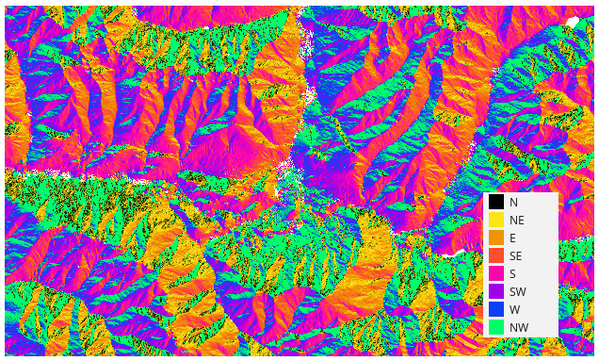Fig. 23.9 Aspect layer reclassified

### 23.1.10.1.1. Parâmetros¶

Etiqueta

Nome

Tipo

Descrição

Elevation layer

`ENTRADA`

[raster]

Digital Terrain Model raster layer

Z factor

`Z_FACTOR`

[number]

Vertical exaggeration. This parameter is useful when the Z units differ from the X and Y units, for example feet and meters. You can use this parameter to adjust for this. The default is 1 (no exaggeration).

Aspecto

`SAÍDA`

[raster]

Specify the output aspect raster layer. One of:

• Save to a Temporary Layer (`TEMPORARY_OUTPUT`)

• Salvar para Arquivo…

A codificação do arquivo também pode ser alterada aqui.

### 23.1.10.1.2. Saídas¶

Etiqueta

Nome

Tipo

Descrição

Aspecto

`SAÍDA`

[raster]

The output aspect raster layer

### 23.1.10.1.3. Python code¶

Algorithm ID: `qgis:aspect`

```import processing
processing.run("algorithm_id", {parameter_dictionary})
```

The algorithm id is displayed when you hover over the algorithm in the Processing Toolbox. The parameter dictionary provides the parameter NAMEs and values. See Usando os algoritmos do processamento a partir do Terminal Python. for details on how to run processing algorithms from the Python console.

## 23.1.10.2. Sombreamento¶

Calculates the hillshade raster layer given an input Digital Terrain Model.

The shading of the layer is calculated according to the sun position: you have the options to change both the horizontal angle (azimuth) and the vertical angle (sun elevation) of the sun.

The hillshade layer contains values from 0 (complete shadow) to 255 (complete sun). Hillshade is used usually to better understand the relief of the area.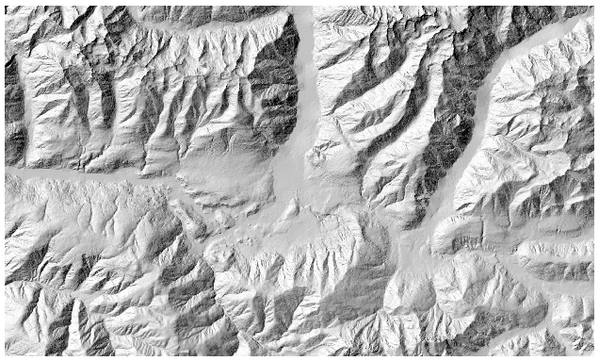Fig. 23.11 Hillshade layer with azimuth 300 and vertical angle 45

Particularly interesting is to give the hillshade layer a transparency value and overlap it with the elevation raster: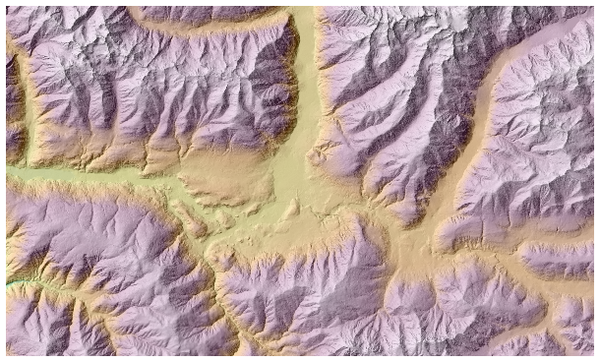Fig. 23.12 Overlapping the hillshade with the elevation layer

### 23.1.10.2.1. Parâmetros¶

Etiqueta

Nome

Tipo

Descrição

Elevation layer

`ENTRADA`

[raster]

Digital Terrain Model raster layer

Z factor

`Z_FACTOR`

[number]

Vertical exaggeration. This parameter is useful when the Z units differ from the X and Y units, for example feet and meters. You can use this parameter to adjust for this. Increasing the value of this parameter will exaggerate the final result (making it look more “hilly”). The default is 1 (no exaggeration).

Azimuth (horizontal angle)

`AZIMUTH`

[number]

Default: 300.0

Set the horizontal angle (in degrees) of the sun (clockwise direction). Range: 0 to 360. 0 is north.

Vertical angle

`V_ANGLE`

[number]

Default: 40.0

Set the vertical angle (in degrees) of the sun, that is the height of the sun. Values can go from 0 (minimum elevation) to 90 (maximum elevation).

Sombreamento

`SAÍDA`

[raster]

Specify the output hillshade raster layer. One of:

• Save to a Temporary Layer (`TEMPORARY_OUTPUT`)

• Salvar para Arquivo…

A codificação do arquivo também pode ser alterada aqui.

Etiqueta

Nome

Tipo

Descrição

Sombreamento

`SAÍDA`

[raster]

### 23.1.10.2.3. Python code¶

Algorithm ID: `qgis:hillshade`

```import processing
processing.run("algorithm_id", {parameter_dictionary})
```

The algorithm id is displayed when you hover over the algorithm in the Processing Toolbox. The parameter dictionary provides the parameter NAMEs and values. See Usando os algoritmos do processamento a partir do Terminal Python. for details on how to run processing algorithms from the Python console.

## 23.1.10.3. Hypsometric curves¶

Calculates hypsometric curves for an input Digital Elevation Model. Curves are produced as CSV files in an output folder specified by the user.

A hypsometric curve is a cumulative histogram of elevation values in a geographical area.

You can use hypsometric curves to detect differences in the landscape due to the geomorphology of the territory.

### 23.1.10.3.1. Parâmetros¶

Etiqueta

Nome

Tipo

Descrição

DEM to analyze

`INPUT_DEM`

[raster]

Digital Terrain Model raster layer to use for calculating altitudes

Boundary layer

`BOUNDARY_LAYER`

[vector: polygon]

Polygon vector layer with boundaries of areas used to calculate hypsometric curves

Step

`STEP`

[number]

Vertical distance between curves

Use % of area instead of absolute value

`USE_PERCENTAGE`

[boolean]

Write area percentage to “Area” field of the CSV file instead of the absolute area

Hypsometric curves

`OUTPUT_DIRECTORY`

[folder]

Specify the output folder for the hypsometric curves. One of:

• Save to a Temporary Layer (`TEMPORARY_OUTPUT`)

• Salvar para Arquivo…

A codificação do arquivo também pode ser alterada aqui.

### 23.1.10.3.2. Saídas¶

Etiqueta

Nome

Tipo

Descrição

Hypsometric curves

`OUTPUT_DIRECTORY`

[folder]

Directory containing the files with the hypsometric curves. For each feature from the input vector layer, a CSV file with area and altitude values will be created.

The file names start with `histogram_`, followed by layer name and feature ID.

### 23.1.10.3.3. Python code¶

Algorithm ID: `qgis:hypsometriccurves`

```import processing
processing.run("algorithm_id", {parameter_dictionary})
```

The algorithm id is displayed when you hover over the algorithm in the Processing Toolbox. The parameter dictionary provides the parameter NAMEs and values. See Usando os algoritmos do processamento a partir do Terminal Python. for details on how to run processing algorithms from the Python console.

## 23.1.10.4. Relief¶

Creates a shaded relief layer from digital elevation data. You can specify the relief color manually, or you can let the algorithm choose automatically all the relief classes.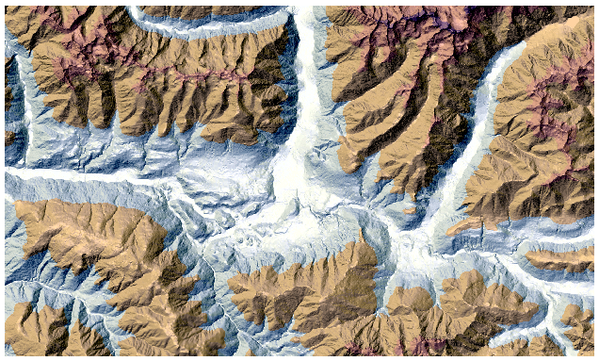Fig. 23.13 Relief layer

### 23.1.10.4.1. Parâmetros¶

Etiqueta

Nome

Tipo

Descrição

Elevation layer

`ENTRADA`

[raster]

Digital Terrain Model raster layer

Z factor

`Z_FACTOR`

[number]

Vertical exaggeration. This parameter is useful when the Z units differ from the X and Y units, for example feet and meters. You can use this parameter to adjust for this. Increasing the value of this parameter will exaggerate the final result (making it look more “hilly”). The default is 1 (no exaggeration).

Generate relief classes automatically

`AUTO_COLORS`

[boolean]

If you check this option the algorithm will create all the relief color classes automatically

Relief colors

Opcional

`COLORS`

[table widget]

Use the table widget if you want to choose the relief colors manually. You can add as many color classes as you want: for each class you can choose the lower and upper bound and finally by clicking on the color row you can choose the color thanks to the color widget.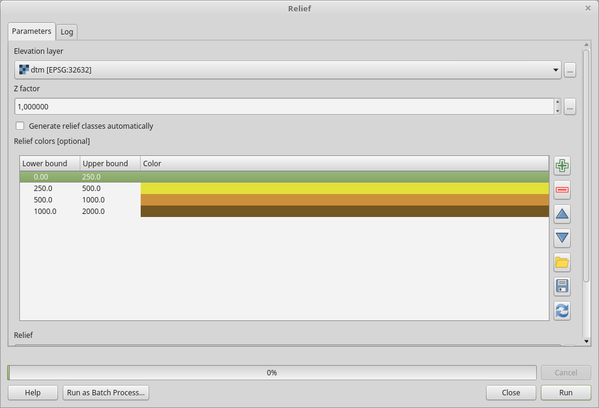Fig. 23.14 Manually setting of relief color classes

The buttons in the right side panel give you the chance to: add or remove color classes, change the order of the color classes already defined, open an existing file with color classes and save the current classes as file.

Relief

`SAÍDA`

[raster]

Default: `[Save to temporary file]`

Specify the output relief raster layer. One of:

• Save to a Temporary Layer (`TEMPORARY_OUTPUT`)

• Salvar para Arquivo…

A codificação do arquivo também pode ser alterada aqui.

Frequency distribution

`FREQUENCY_DISTRIBUTION`

[table]

Default: `[Skip output]`

Specify the CSV table for the output frequency distribution. One of:

• Ignorar Saída

• Save to a Temporary Layer (`TEMPORARY_OUTPUT`)

• Salvar para Arquivo…

A codificação do arquivo também pode ser alterada aqui.

### 23.1.10.4.2. Saídas¶

Etiqueta

Nome

Tipo

Descrição

Relief

`SAÍDA`

[raster]

The output relief raster layer

Frequency distribution

`SAÍDA`

[table]

The output frequency distribution

### 23.1.10.4.3. Python code¶

Algorithm ID: `qgis:relief`

```import processing
processing.run("algorithm_id", {parameter_dictionary})
```

The algorithm id is displayed when you hover over the algorithm in the Processing Toolbox. The parameter dictionary provides the parameter NAMEs and values. See Usando os algoritmos do processamento a partir do Terminal Python. for details on how to run processing algorithms from the Python console.

## 23.1.10.5. Ruggedness index¶

Calculates the quantitative measurement of terrain heterogeneity described by Riley et al. (1999). It is calculated for every location, by summarizing the change in elevation within the 3x3 pixel grid.

Each pixel contains the difference in elevation from a center cell and the 8 cells surrounding it.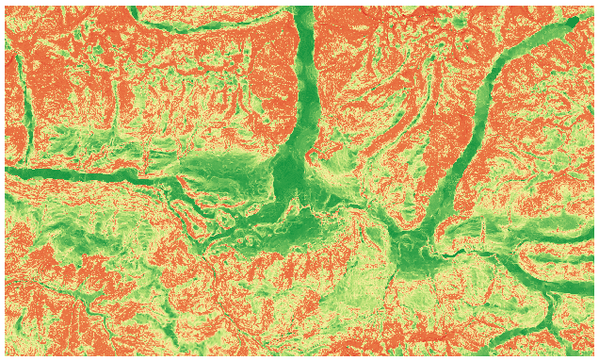Fig. 23.15 Ruggedness layer from low (red) to high values (green)

### 23.1.10.5.1. Parâmetros¶

Etiqueta

Nome

Tipo

Descrição

Elevation layer

`ENTRADA`

[raster]

Digital Terrain Model raster layer

Z factor

`Z_FACTOR`

[number]

Vertical exaggeration. This parameter is useful when the Z units differ from the X and Y units, for example feet and meters. You can use this parameter to adjust for this. Increasing the value of this parameter will exaggerate the final result (making it look more rugged). The default is 1 (no exaggeration).

Ruggedness

`SAÍDA`

[raster]

Default: `[Save to temporary file]`

Specify the output ruggedness raster layer. One of:

• Save to a Temporary Layer (`TEMPORARY_OUTPUT`)

• Salvar para Arquivo…

A codificação do arquivo também pode ser alterada aqui.

### 23.1.10.5.2. Saídas¶

Etiqueta

Nome

Tipo

Descrição

Ruggedness

`SAÍDA`

[raster]

The output ruggedness raster layer

### 23.1.10.5.3. Python code¶

Algorithm ID: `qgis:ruggednessindex`

```import processing
processing.run("algorithm_id", {parameter_dictionary})
```

The algorithm id is displayed when you hover over the algorithm in the Processing Toolbox. The parameter dictionary provides the parameter NAMEs and values. See Usando os algoritmos do processamento a partir do Terminal Python. for details on how to run processing algorithms from the Python console.

## 23.1.10.6. Pendiente¶

Calculates the slope from an input raster layer. The slope is the angle of inclination of the terrain and is expressed in degrees.

In the following picture you can see to the left the DTM layer with the elevation of the terrain while to the right the calculated slope: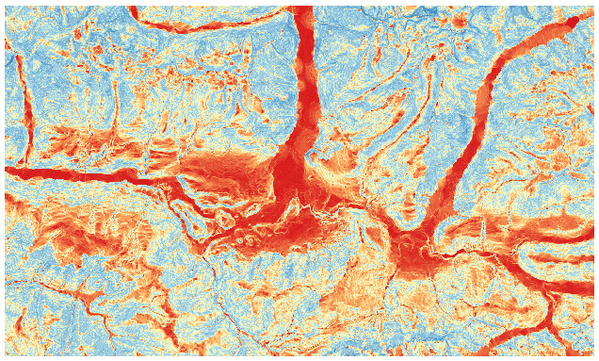Fig. 23.16 Flat areas in red, steep areas in blue

### 23.1.10.6.1. Parâmetros¶

Etiqueta

Nome

Tipo

Descrição

Elevation layer

`ENTRADA`

[raster]

Digital Terrain Model raster layer

Z factor

`Z_FACTOR`

[number]

Vertical exaggeration. This parameter is useful when the Z units differ from the X and Y units, for example feet and meters. You can use this parameter to adjust for this. Increasing the value of this parameter will exaggerate the final result (making it steeper). The default is 1 (no exaggeration).

Slope

`SAÍDA`

[raster]

Default: `[Save to temporary file]`

Specify the output slope raster layer. One of:

• Save to a Temporary Layer (`TEMPORARY_OUTPUT`)

• Salvar para Arquivo…

A codificação do arquivo também pode ser alterada aqui.

### 23.1.10.6.2. Saídas¶

Etiqueta

Nome

Tipo

Descrição

Slope

`SAÍDA`

[raster]

The output slope raster layer

### 23.1.10.6.3. Python code¶

Algorithm ID: `qgis:slope`

```import processing
processing.run("algorithm_id", {parameter_dictionary})
```

The algorithm id is displayed when you hover over the algorithm in the Processing Toolbox. The parameter dictionary provides the parameter NAMEs and values. See Usando os algoritmos do processamento a partir do Terminal Python. for details on how to run processing algorithms from the Python console.